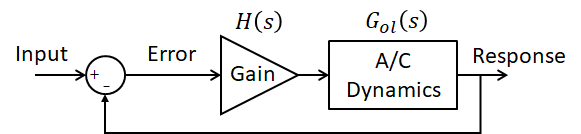Servers by jDrones# Predicting Closed Loop Response For Faster Autotune

As you may know I have been working for quite some time on Heli autotune. It has been journey of learning, first on how to do this in the frequency domain and then what makes a good tune. I have had the opportunity to working on a manned helicopter program where we looked to predict the feedback gain (what would be the PID rate P gain) that would maintain 6 db gain margin to the instability. I worked from analysis that was provided to me without truly understanding the underlying theory. Recently I was looking into pilot induced oscillations and came across the same theory that was used to determine the feedback gain. After looking into this this further, I figured out how this theory could be applied to autotune to predict the closed loop response and hopefully minimize the time it takes to tune vehicles.

First let me explain open loop and closed loop. Open loop is when the vehicles response is not being used to modify its control. It would be like flying an airplane with no stabilizing electronics. Essentially using only the RC receiver to make inputs into the control surfaces. Closed loop is when the vehicle response (angular rate, attitude) are used to help stabilize the vehicle. Here is a diagram of a simple control system where the aircraft response is fed back into the control of the vehicle (closed loop system)The open loop is between the error and the response where as the closed loop is between the input and the response.

We can use a nichols plot to move between the open loop response and the closed loop response for this simple system. The nichols plot for the yaw axis of a helicopter is shown below

The blue dots are the frequency response of the yaw axis. Each point is a dwell at a frequency and the resulting open loop response gain and phase is plotted. The lines overlaid on the plot are lines of constant closed loop response gain. You can see the 0 db and 3 db closed loop response lines. It shows that the current Rate P gain setting of 0.2 has the closed loop response peak gain a little greater than 0 db, maybe 1 db. With this plot, I can now predict the Rate P gain necessary to achieve a closed loop gain of 3 db by measuring the change in gain between the data and the 3 db closed loop line. Changing the Rate P gain just moves this data up and down on the plot. So you just want it to touch the edge of the 3 db line.

Thus we increase the Rate P gain by 1.35 db which is multiplying it by 1.16 and the new rate P gain would be 0.232. Conducting dwells at several frequencies and plotting the resulting data you get

You can see that the closed loop response for the new Rate P gain touches the 3 db line

This simple close loop system is identical to the attitude controller in Copter, except the rate controller is in there and would look like thisTherefore this analysis technique will work well for the tuning the angle P gain. I believe it will still work well for the Rate P gain too. For helicopter’s pitch and roll axes where the control of the vehicle relies primarily on FF, this becomes a little more difficult for the Rate P gain. I believe it can still be predicted but relies on the FF value. If the FF gain changes then it would affect the rate P prediction. In all aircraft, the Angle P gain can definitely be predicted using this technique.
Maybe I can get someone on the Plane side to look into implementing this to test it out.

7 Likes

Bill, how many cycles at each frequency point are you using to get the required magnitude and phase accuracy?

@priseborough I am using five cycles to allow it to reach steady state and then another five cycles to determine the gain and phase. Those five cycles are averaged and I don’t do any calculations of the confidence of the prediction. The data that I’ve reviewed thus far has shown pretty consistent magnitude and phase across those five cycles.

Now that you bring that up, it may not be a bad idea to use something like a confidence interval to determine when a noisy signal is affecting the calculation. Maybe require estimate to be within 5% with 90% confidence? I suppose that requires that I know what the answer should be. So maybe looking at variability below a certain value to say it is good?

Servers by jDrones